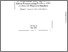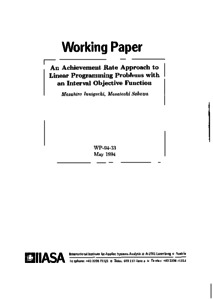# An Achievement Rate Approach to Linear Programming Problems with an Interval Objective Function

Inuiguchi, M. & Sakawa, M. (1994). An Achievement Rate Approach to Linear Programming Problems with an Interval Objective Function. IIASA Working Paper. IIASA, Laxenburg, Austria: WP-94-033Preview
Text
WP-94-033.pdf

## Abstract

In this paper, we focus on a treatment of a linear programming problem with an interval objective function. From the viewpoint of the achievement rate, a new solution concept, a maximin achievement rate solution is proposed. Nice properties of this solution are shown: a maximin achievement rate solution is necessarily optimal when a necessarily optimal solution exists, and if not, then it is still a possibly optimal solution. An algorithm for a maximin achievement rate solution is proposed based on a relaxation procedure together with a simplex method. A numerical example is given to demonstrate the proposed solution algorithm.

Item Type: Monograph (IIASA Working Paper) Methodology of Decision Analysis (MDA) IIASA Import 15 Jan 2016 02:04 27 Aug 2021 17:14 http://pure.iiasa.ac.at/4176View Item